# Periodically Amplified Lightwave Systems

Many experiments performed during the early 1990s employed a chain of cascaded in-line optical amplifiers for increasing the length of long-haul fiber links. These experiments showed that fiber dispersion becomes the limiting factor in periodically amplified lightwave systems. Indeed, the experiments were feasible only because the system was operated close to the zero-dispersion wavelength of the fiber link. Moreover, the residual dispersion was tailored along the link in such a way that the total dispersion over the entire link length was quite small at the operating wavelength. By 1992, total system length could be increased to beyond 10,000 km with such dispersion-management techniques. In a 1991 experiment, an effective transmission distance of 21,000 km at 2.5 Gb/s and of 14,300 km at 5 Gb/s was realized using a recirculating fiber loop. By 2010, 96 channels, each channel operating at 100 Gb/s, could be transmitted over 10,600 km by using a phase-based modulation format.

A rough estimate of dispersion-limited system length can be obtained if launched power is assumed to be low enough that one can neglect the nonlinear effects during signal transmission. Since amplifiers compensate only for fiber losses, dispersion limitations discussed in this tutorial and shown in the following figure apply for each channel of a WDM system if L is replaced by LT.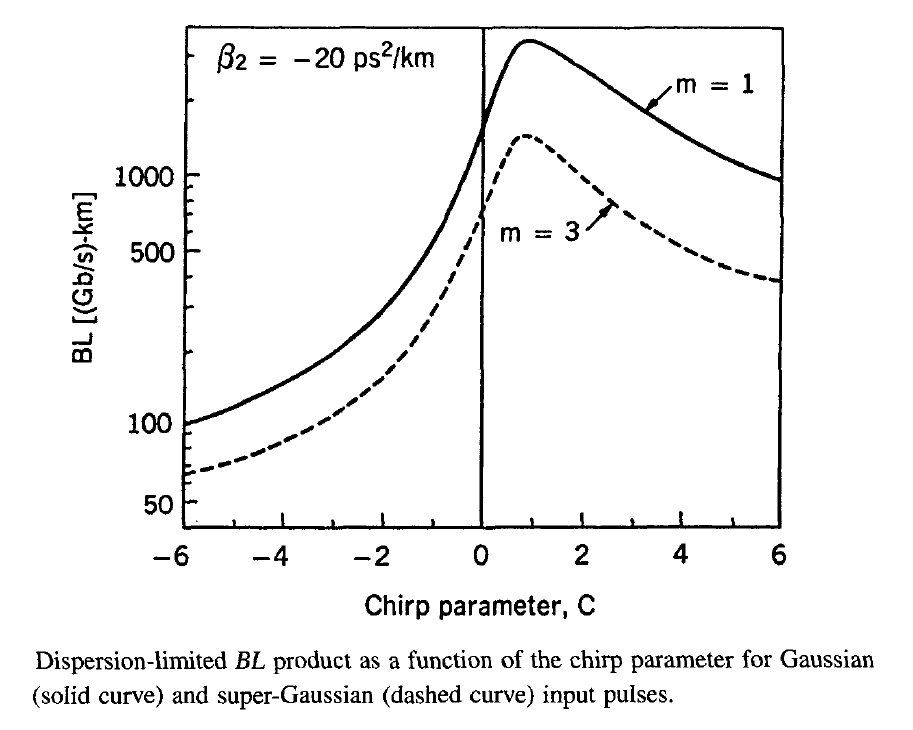From the following equation,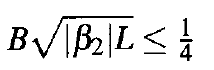the dispersion limit for systems making use of standard fibers (β2 ≈ -20 ps2/km at 1.55 μm) is B2L < 3,000 (Gb/s)2-km. Such a system length is limited to below 2 km at 40 Gb/s and this length increases to only 30 km at 10 Gb/s. An increase by a factor of 20 can be realized by using dispersion-shifted fibers with |β2| ≈ 1 ps2/km. To extend the distance to beyond 6,000 km at 10 Gb/s, average GVD along the link should be smaller than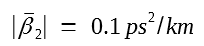#### 1. Numerical Approach

The preceding estimate is crude since it does not include the impact of nonlinear effects discussed in this tutorial. Even though power levels are kept relatively modest for each channel, the nonlinear effects can become quite important because of their accumulation over long distances. For single-channel systems, the most dominant nonlinear phenomenon that limits the system performance is SPM. An estimate of power limitation imposed by the SPM can be obtained from the following equation:If we use α = 0.2 dB/km and γ = 2 W-1/km as typical values, and assume that the fiber link contains 100 amplifiers with 50-km spacing, the input power should be below 0.25 mW (-6 dBm) for the resulting 5,000-km-long system. Such power levels may not be high enough to maintain the required Q factor when ASE noise accumulated over 100 amplifiers is taken into account. The only solution would then be to decrease the number of the cascaded amplifiers.

An analytic estimate of the SPM-limited distance provides only a rough guideline as it ignores not only fiber dispersion but also the accumulation of ASE noise along the link. In reality, the nonlinear and dispersive effects act on a noisy optical signal simultaneously, and their mutual interplay is quite important. For this reason, the most practical approach for designing modern lightwave systems consists of solving the NLS equation below directly using a suitable numerical technique.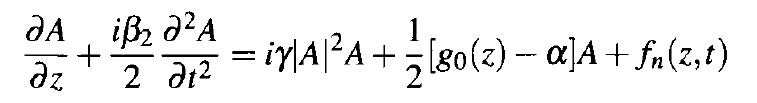Numerical simulations indeed show that the accumulation of the nonlinear effects often limits the system length in practical lightwave systems and requires optimization of various design parameters such as amplifier spacing, input power launched into fiber, and dispersion properties of fibers used to construct the transmission link. Indeed, several software packages dealing with the design of lightwave systems are available commercially. One such package is marketed by Optiwave and called OptiSystem.

The main advantage of a computer-aided design technique for designing modern lightwave systems is that such an approach is capable of optimizing the whole system and can provide the optimum values of various system parameters such that the design objectives are met at a minimum cost. The following figure illustrates the various steps involved in the simulation process. The approach consists of generating an optical bit pattern at the transmitter, transmitting it through the fiber link, detecting it at the receiver, and then analyzing it through the tools such as the eye diagram and the Q factor.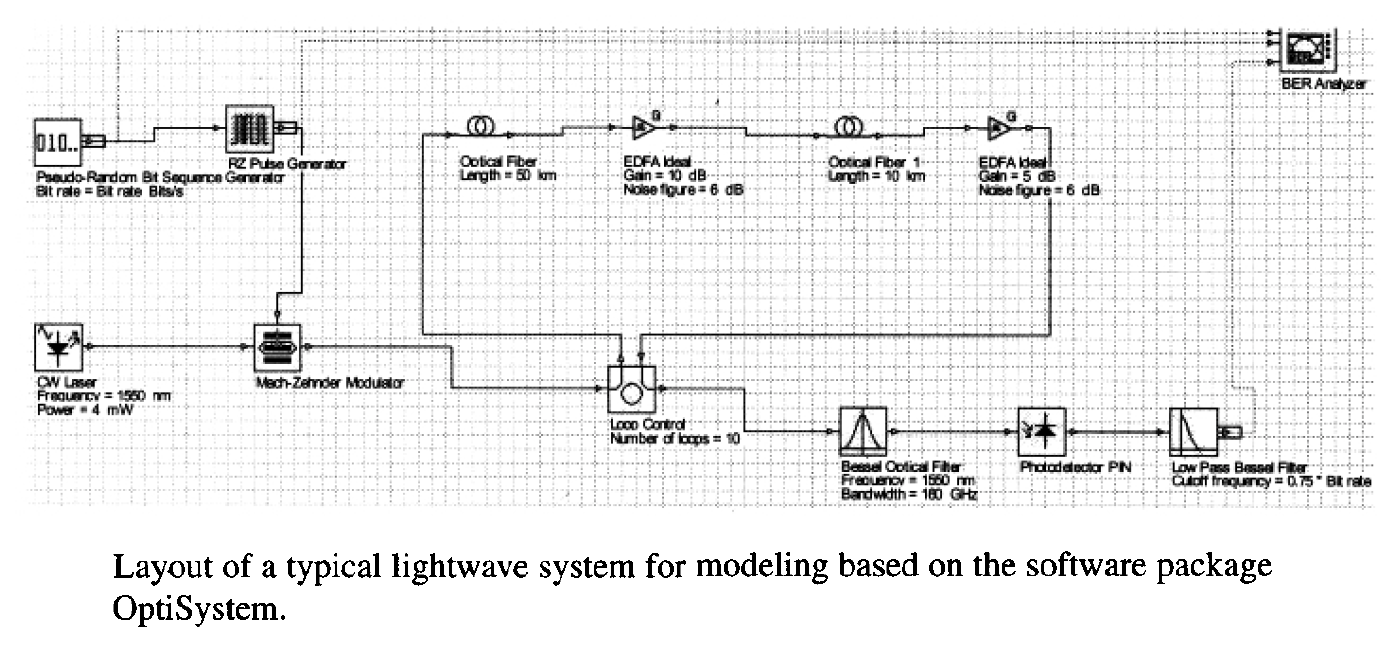At the transmitter end, a pseudorandom bit sequence (PRBS) is used to create an RZ or NRZ electrical bit stream. The length N of the bit stream determines the computing time and should be chosen judiciously. Typically, N = 2M, where M is in the range of 6 to 10. The optical bit steam is obtained by solving the rate equations that govern the modulation response of a semiconductor laser, if direct modulation is employed. A different set of equations governing the dynamics of an external modulator should be used if such a device is used to convert the CW laser light into an optical bit stream. Chirping of optical pulses is automatically included in both cases. Deformation of the optical bit stream during its transmission through the optical fiber is calculated by solving the NLS equation. The method most commonly used for solving this equation is known as the spit-step Fourier method.

The noise added by optical amplifiers should be included in any realistic simulation. In the case of lumped amplifiers, the NLS equation is solved without the gain and noise terms in each fiber section, and ASE noise is added to the signal at the location of each amplifier through the following equation:In the case of distributed amplification, noise must be added throughout the fiber length and it should satisfy the correlation function given byThere are two equivalent techniques for adding ASE noise to the signal during numerical simulations.

In one case, noise is added in the time domain, while ensuring that it follows Gaussian statistics and has a spectral density given byin the lumped case,

and given byin the distributed case.

In an alternative approach, noise is added in the frequency domain. In the case of lumped amplifiers, the spectral amplitude at the amplifier output is given bywhere a tilde represents the Fourier transform. The noise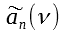is assumed to be frequency-independent (white noise) over the whole amplifier bandwidth, or the filter bandwidth if an optical filter is used after each amplifier. Mathematically,is a complex Gaussian random variable whose real and imaginary parts have the spectral density SASE/2.

After adding noise at each amplifier, the NLS equation is solved in the following fiber section, and the procedure is repeated until the last amplifier is reached. A suitable receiver model converts optical signal into the electric domain and filters it using a filter whose bandwidth Δf is close to but smaller than the bit rate B (typically Δf/B = 0.6-0.8). The resulting electric bit stream is used to find the instantaneous values of currents, I0 and I1, for 0 and 1 bits, respectively, by sampling it at the center of each bit slot. An eye diagram is also constructed using the filtered bit stream. The system performance is quantified through the Q factor, defined below: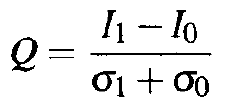and related directly to the BER through this equation: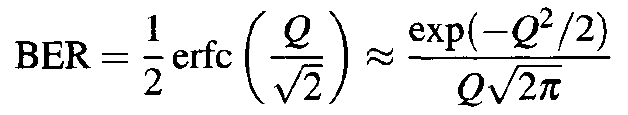The calculation of the Q factor requires that the NLS equation be solved a large number of times with different seeds for the amplifier noise. Such an approach can be used to investigate trade-offs that would optimize overall system performance.

Computer-aided design has another important role to play. A long-haul lightwave system may contain many repeaters, both optical and electrical. Transmitters, receivers, and amplifiers used at repeaters, although chosen to satisfy nominal specifications, are never identical. Similarly, fiber cables are constructed by splicing many different pieces (with a typical length of 4 to 8 km) that have slightly different loss and dispersion characteristics. The net result is that many system parameters vary around their nominal values. For example, the dispersion parameter D, responsible not only for pulse broadening but also for other sources of power penalty, can vary significantly in different sections of the fiber link because of variations in the zero-dispersion wavelength and the transmitter wavelength. A statistical approach is often used to estimate the effect of such inherent variations on the performance of a realistic lightwave system. The idea behind such an approach is that it is extremely unlikely that all system parameters would take their worst-case values at the same time. Thus, repeater spacing can be increased well above its worst-case value if the system is designed to operate reliably at the specific bit rate with a high probability (say, 99.9%).

#### 2. Optimum Launched Power

The importance of computer-aided design for fiber-optic communication systems became apparent during the 1990s with the advent of optical amplifiers. Amplifiers not only added ASE noise to the signal but also allow the dispersive and nonlinear effects to accumulate over long lengths. Moreover, amplifier noise often forces one to increase the channel power to more than 1 mW in order to maintain a high SNR (and a high Q factor consistent with the BER requirements). Since noise limits the Q factor at low power levels whereas nonlinear effects limit it at high power levels, it is evident that a lightwave system would have the largest value of Q at an optimum value of the average power launched into the fiber at the input end. The following figure shows schematically how the Q factor varies with the average input power for a long-haul lightwave system limited by the nonlinear effects. The Q factor increases initially with launched power, reaches a peak value, and then decreases with a further increase in the power because of the onset of the nonlinear effects.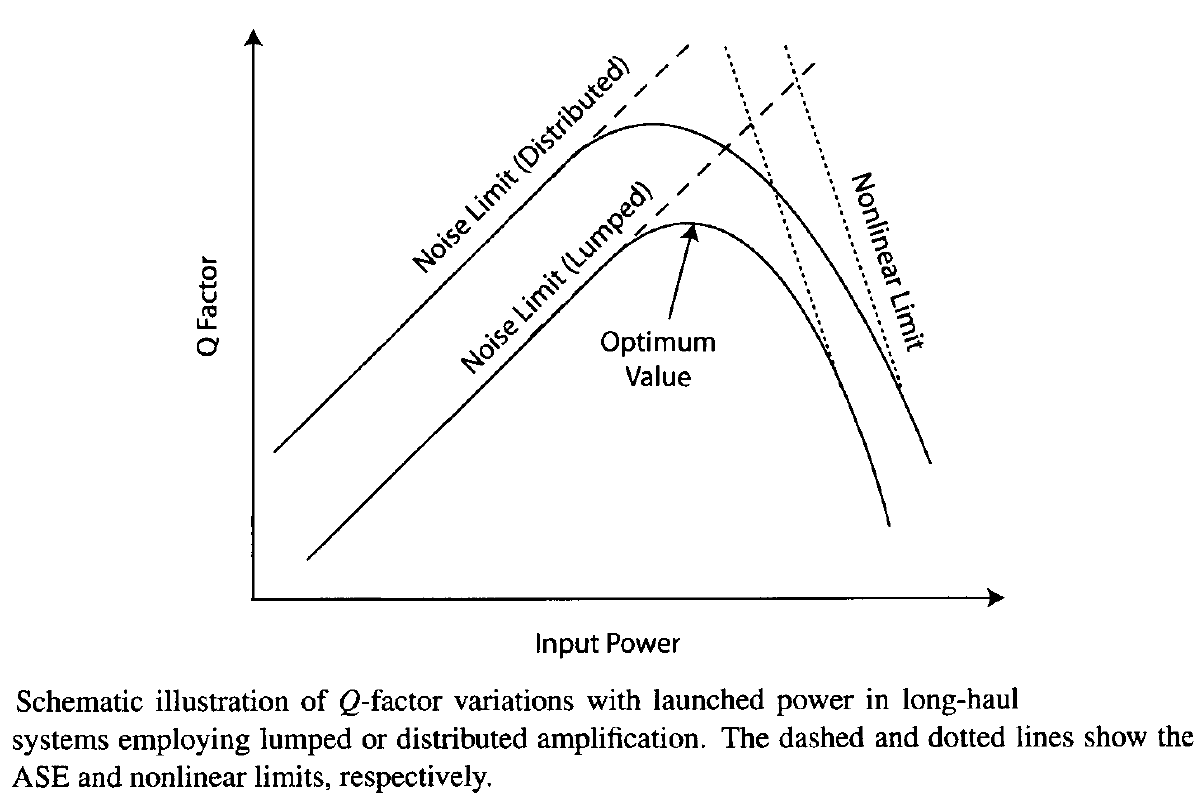The figure above also illustrates why the use of distributed amplification, in place of lumped amplifiers, improves system performance. Since the level of ASE noise is lower in the case of distributed amplification, such systems exhibit a higher optical SNR (or the Q factor) at a given power level (dashed line). As a result, the power level at which Q peaks is smaller compared with the lumped case. From a practical perspective, one can trade off a higher Q with a larger transmission distance. In other words, distributed amplification helps to increase the link length.

This feature is shown in the following figure, where numerical results are shown for a 32-channel WDM system by plotting the maximum possible transmission distance as a function of input power assuming that a value of Q = 7 must be maintained at the end to ensure a BER below 10-12. The fiber link is divided into 80-km sections, and the 20-dB loss of each section is compensated using a forward or backward pumping configuration.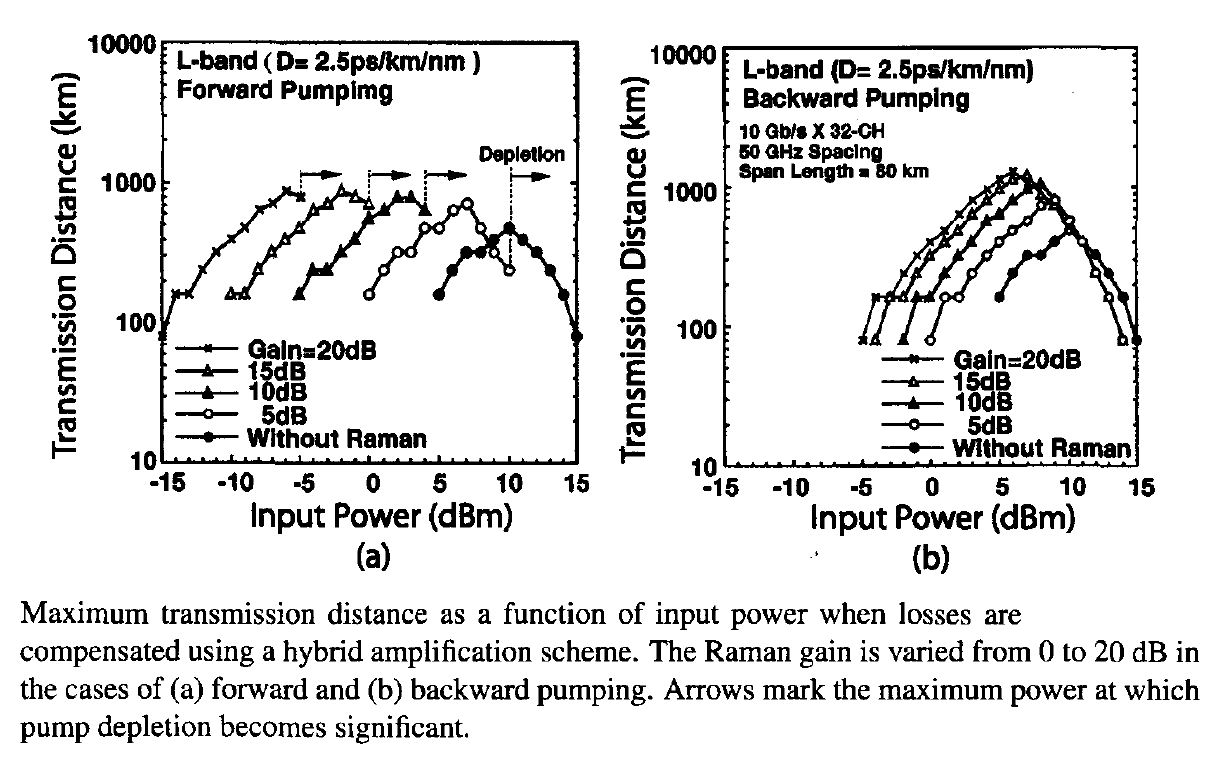Several features of the above figure are noteworthy. As expected from the previous figure, transmission distance is maximum at an optimum value of input power. This optimum value is largest (about 10 mW), and the distance is smallest (about 480 km) in the case of pure lumped amplification. As the amount of Raman gain increases, the optimum power is reduced and transmission distance increases. The same signal can be transmitted over more than 1,000 km when all losses are compensated through Raman amplification. The optimum value of input power drops below -5 dBm in the case of forward pumping. The more than two-fold increase in the link length with the use of Raman amplification is due to a reduced ASE noise that is added to the signal during its transmission.

Some terrestrial systems serving the backbone of a wide-area network should operate over more than 3,000 km (called ultra-long-haul systems). This can be realized by employing forward error correction because the minimum value of Q required at the receiver then drops to close to 3 (rather than being 7, as assumed in the last figure). Indeed, by 2004, a WDM system with 128 channels, each channel operating at 10 Gb/s, was able to transmit information over 4,000 km when it was designed to compensate losses through forward Raman pumping in each 100-km section of dispersion-shifted fibers. Even when standard fibers were employed, the system could be operated over 3,200 km with a pump-station spacing of 80 km. In both cases, it was necessary to employ forward error correction with 7% overhead. The average power launched into the fiber link was only -5 dBm. Such low power was enough to maintain an optical SNR of more than 15 dB for all channels because of a low noise level associated with distributed Raman amplification in the forward-pumping configuration. It was also important to compensate for fiber dispersion along the fiber link. In general, system performance can be improved with dispersion management. We turn to this issue in the next tutorial.